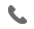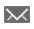•+44 7459 302492info@uplatz.com
• Register
Job Meter = High

### Applied Analytics Using SAS Enterprise Miner

30 Hours
Online Instructor-led Training
USD 2800318 Learners

This course covers the skills that are required to assemble analysis flow diagrams using the rich tool set of SAS Enterprise Miner for both pattern discovery (segmentation, association, and sequence analyses) and predictive modeling (decision tree, regression, and neural network models). This course is appropriate for SAS Enterprise Miner 5.3 up to 15.1.

Learn how to
• Define a SAS Enterprise Miner project and explore data graphically.
• Modify data for better analysis results.
• Build and understand predictive models such as decision trees and regression models.
• Compare and explain complex models.
• Generate and use score code.
• Apply association and sequence discovery to transaction data.
--------------------------------------------------------------
Target Audience

Data analysts, qualitative experts, and others who want an introduction to SAS Enterprise Miner
--------------------------------------------------------------

# Applied Analytics Using SAS Enterprise Miner

Course Details & Curriculum
Introduction
• Introduction to SAS Enterprise Miner.
Accessing and Assaying Prepared Data
• Creating a SAS Enterprise Miner project, library, and diagram.
• Defining a data source.
• Exploring a data source.
Introduction to Predictive Modeling: Predictive Modeling Fundamentals and Decision Trees
• Introduction.
• Cultivating decision trees.
• Optimizing the complexity of decision trees.
• Understanding additional diagnostic tools (self-study).
• Autonomous tree growth options (self-study).
Introduction to Predictive Modeling: Regressions
• Selecting regression inputs.
• Optimizing regression complexity.
• Interpreting regression models.
• Transforming inputs.
• Categorical inputs.
• Polynomial regressions (self-study).
Introduction to Predictive Modeling: Neural Networks and Other Modeling Tools
• Input selection.
• Stopped training.
• Other modeling tools (self-study).
Model Assessment
• Model fit statistics.
• Statistical graphics.
• Profit matrices.
Model Implementation
• Internally scored data sets.
• Score code modules.
Introduction to Pattern Discovery
• Cluster analysis.
Special Topics
• Ensemble models.
• Variable selection.
• Categorical input consolidation.
• Surrogate models.
• SAS Rapid Predictive Modeler.
Case Studies
• Banking segmentation case study.
• Website usage associations case study.
• Credit risk case study.
• Enrollment management case study.
--------------------------------------------------------------